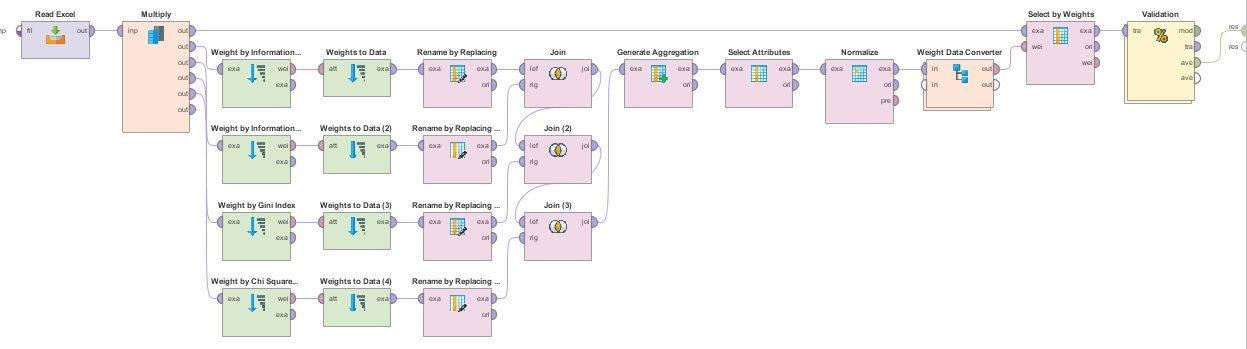# Optimize selection

Member Posts: 17Contributor I
edited December 2018 in Help

Hi

I need your guide and i hope there is someone that help me.

Actually I have 13 features in my project and I need the features that have the highest percentage of accuracyI used a feature reduction feature using different weights, for example, I set the parameter k to 5 and the 5 features that had the highest weight were considered for accuracy checking.But this method is not responsive and the accuracy does not go up. So, I decided to change my method.i need Optimize Selection Operator to examine all the features and consider the features that will increase the accuracy. can you send me a sample of how to use this Operator? i am sending a picture of Feature Reduction method based on different weights that i perform in my project.best regard

m.gh• Moderator, RapidMiner Certified Analyst, Member Posts: 1,195Unicorn
Solution Accepted

Here an example of process using the Optimize Selection (Evolutionary) operator :

`<?xml version="1.0" encoding="UTF-8"?><process version="8.2.001">  <context>    <input/>    <output/>    <macros/>  </context>  <operator activated="true" class="process" compatibility="8.2.001" expanded="true" name="Process">    <process expanded="true">      <operator activated="true" class="retrieve" compatibility="8.2.001" expanded="true" height="68" name="Retrieve Sonar" width="90" x="112" y="85">        <parameter key="repository_entry" value="//Samples/data/Sonar"/>      </operator>      <operator activated="true" class="concurrency:cross_validation" compatibility="8.2.001" expanded="true" height="145" name="Cross Validation" width="90" x="380" y="85">        <parameter key="number_of_folds" value="5"/>        <process expanded="true">          <operator activated="true" class="multiply" compatibility="8.2.001" expanded="true" height="103" name="Multiply" width="90" x="45" y="34"/>          <operator activated="true" class="remember" compatibility="8.2.001" expanded="true" height="68" name="Remember" width="90" x="179" y="34">            <parameter key="name" value="trainingSet"/>          </operator>          <operator activated="true" class="optimize_selection_evolutionary" compatibility="8.2.001" expanded="true" height="103" name="Optimize Selection (Evolutionary)" width="90" x="179" y="187">            <process expanded="true">              <operator activated="true" class="concurrency:cross_validation" compatibility="8.2.001" expanded="true" height="145" name="Cross Validation (2)" width="90" x="246" y="34">                <parameter key="number_of_folds" value="5"/>                <process expanded="true">                  <operator activated="true" class="naive_bayes" compatibility="8.2.001" expanded="true" height="82" name="Naive Bayes (2)" width="90" x="246" y="34"/>                  <connect from_port="training set" to_op="Naive Bayes (2)" to_port="training set"/>                  <connect from_op="Naive Bayes (2)" from_port="model" to_port="model"/>                  <portSpacing port="source_training set" spacing="0"/>                  <portSpacing port="sink_model" spacing="0"/>                  <portSpacing port="sink_through 1" spacing="0"/>                </process>                <process expanded="true">                  <operator activated="true" class="apply_model" compatibility="8.2.001" expanded="true" height="82" name="Apply Model (2)" width="90" x="112" y="34">                    <list key="application_parameters"/>                  </operator>                  <operator activated="true" class="performance_classification" compatibility="8.2.001" expanded="true" height="82" name="Performance (2)" width="90" x="246" y="34">                    <list key="class_weights"/>                  </operator>                  <connect from_port="model" to_op="Apply Model (2)" to_port="model"/>                  <connect from_port="test set" to_op="Apply Model (2)" to_port="unlabelled data"/>                  <connect from_op="Apply Model (2)" from_port="labelled data" to_op="Performance (2)" to_port="labelled data"/>                  <connect from_op="Performance (2)" from_port="performance" to_port="performance 1"/>                  <connect from_op="Performance (2)" from_port="example set" to_port="test set results"/>                  <portSpacing port="source_model" spacing="0"/>                  <portSpacing port="source_test set" spacing="0"/>                  <portSpacing port="source_through 1" spacing="0"/>                  <portSpacing port="sink_test set results" spacing="0"/>                  <portSpacing port="sink_performance 1" spacing="0"/>                  <portSpacing port="sink_performance 2" spacing="0"/>                </process>              </operator>              <connect from_port="example set" to_op="Cross Validation (2)" to_port="example set"/>              <connect from_op="Cross Validation (2)" from_port="performance 1" to_port="performance"/>              <portSpacing port="source_example set" spacing="0"/>              <portSpacing port="source_through 1" spacing="0"/>              <portSpacing port="sink_performance" spacing="0"/>            </process>          </operator>          <operator activated="true" class="select_by_weights" compatibility="8.2.001" expanded="true" height="103" name="Select by Weights" width="90" x="313" y="34"/>          <operator activated="true" class="naive_bayes" compatibility="8.2.001" expanded="true" height="82" name="Naive Bayes" width="90" x="447" y="34"/>          <connect from_port="training set" to_op="Multiply" to_port="input"/>          <connect from_op="Multiply" from_port="output 1" to_op="Remember" to_port="store"/>          <connect from_op="Multiply" from_port="output 2" to_op="Optimize Selection (Evolutionary)" to_port="example set in"/>          <connect from_op="Remember" from_port="stored" to_op="Select by Weights" to_port="example set input"/>          <connect from_op="Optimize Selection (Evolutionary)" from_port="weights" to_op="Select by Weights" to_port="weights"/>          <connect from_op="Select by Weights" from_port="example set output" to_op="Naive Bayes" to_port="training set"/>          <connect from_op="Select by Weights" from_port="weights" to_port="through 1"/>          <connect from_op="Naive Bayes" from_port="model" to_port="model"/>          <portSpacing port="source_training set" spacing="0"/>          <portSpacing port="sink_model" spacing="0"/>          <portSpacing port="sink_through 1" spacing="0"/>          <portSpacing port="sink_through 2" spacing="0"/>        </process>        <process expanded="true">          <operator activated="true" class="select_by_weights" compatibility="8.2.001" expanded="true" height="103" name="Select by Weights (2)" width="90" x="45" y="85"/>          <operator activated="true" class="recall" compatibility="8.2.001" expanded="true" height="68" name="Recall (4)" width="90" x="45" y="238">            <parameter key="name" value="trainingSet"/>          </operator>          <operator activated="true" class="superset" compatibility="8.2.001" expanded="true" height="82" name="Superset" width="90" x="246" y="187"/>          <operator activated="true" class="apply_model" compatibility="8.2.001" expanded="true" height="82" name="Apply Model" width="90" x="246" y="34">            <list key="application_parameters"/>          </operator>          <operator activated="true" class="performance_classification" compatibility="8.2.001" expanded="true" height="82" name="Performance" width="90" x="380" y="34">            <list key="class_weights"/>          </operator>          <connect from_port="model" to_op="Apply Model" to_port="model"/>          <connect from_port="test set" to_op="Select by Weights (2)" to_port="example set input"/>          <connect from_port="through 1" to_op="Select by Weights (2)" to_port="weights"/>          <connect from_op="Select by Weights (2)" from_port="example set output" to_op="Superset" to_port="example set 1"/>          <connect from_op="Recall (4)" from_port="result" to_op="Superset" to_port="example set 2"/>          <connect from_op="Superset" from_port="superset 1" to_op="Apply Model" to_port="unlabelled data"/>          <connect from_op="Apply Model" from_port="labelled data" to_op="Performance" to_port="labelled data"/>          <connect from_op="Performance" from_port="performance" to_port="performance 1"/>          <connect from_op="Performance" from_port="example set" to_port="test set results"/>          <portSpacing port="source_model" spacing="0"/>          <portSpacing port="source_test set" spacing="0"/>          <portSpacing port="source_through 1" spacing="0"/>          <portSpacing port="source_through 2" spacing="0"/>          <portSpacing port="sink_test set results" spacing="0"/>          <portSpacing port="sink_performance 1" spacing="0"/>          <portSpacing port="sink_performance 2" spacing="0"/>        </process>      </operator>      <connect from_op="Retrieve Sonar" from_port="output" to_op="Cross Validation" to_port="example set"/>      <connect from_op="Cross Validation" from_port="model" to_port="result 2"/>      <connect from_op="Cross Validation" from_port="example set" to_port="result 1"/>      <connect from_op="Cross Validation" from_port="test result set" to_port="result 3"/>      <connect from_op="Cross Validation" from_port="performance 1" to_port="result 4"/>      <portSpacing port="source_input 1" spacing="0"/>      <portSpacing port="sink_result 1" spacing="0"/>      <portSpacing port="sink_result 2" spacing="0"/>      <portSpacing port="sink_result 3" spacing="0"/>      <portSpacing port="sink_result 4" spacing="0"/>      <portSpacing port="sink_result 5" spacing="0"/>    </process>  </operator></process>`

The feature selection itself has to be validated, that's why the Optimize Selection (Evolutionary) operator is implemented

inside the "training part" of a Cross Validation operator.

I hope it helps,

Regards,

Lionel